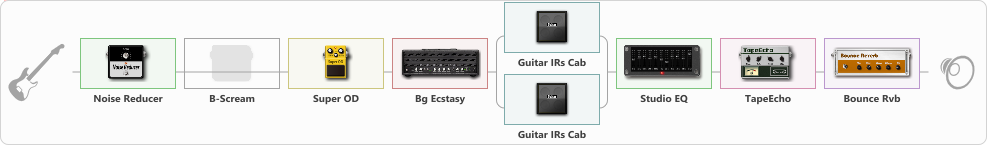# BOGN crunch Truss

Discussion in 'ToneLib-GFX presets' started by truss van halen, Feb 19, 2022.

1. ### truss van halenWell-Known Member

BOGN crunch Truss

Preset name: MY Crunch

Effects chain:Effect: "Noise Reducer" (Dynamics / Filter), active - "yes"
{
"Sens" = 100
"Mode" = Hard
}

Effect: "B-Scream" (Overdrive / Distortion), active - "no"
{
"Drive" = 43
"Tone" = 55
"Level" = 60
}

Effect: "Super OD" (Overdrive / Distortion), active - "yes"
{
"Drive" = 21
"Tone" = 80
"Level" = 91
}

Effect: "Bg Ecstasy" (Amp simulators), active - "yes"
{
"Gain" = 26
"Bass" = 50
"Middle" = 50
"Treble" = 50
"Presence" = 50
"Master" = 64
"Level (dB)" = 12
}

Effect: "Splitter" (Dynamics / Filter)
{
"A-Bypass" = Off
"A-Pan" = 0
"A-Level" = 55
"B-Bypass" = Off
"B-Pan" = 0
"B-Level" = 60
"Width" = 0

'A' branch:
{

Effect: "Guitar IRs Cab" (Cabinets), active - "yes"
{
"Model" = Marshall 1960A (4x12")
"Mic Position" = Middle
"Mic Distance" = Far
"Low Cut (Hz)" = 80
"Hi Cut (kHz)" = 10.0
"Mix" = 100
"Level (dB)" = 0
}
}
'B' branch:
{

Effect: "Guitar IRs Cab" (Cabinets), active - "yes"
{
"Model" = Soldano Slant (4x12")
"Mic Position" = Center
"Mic Distance" = Middle
"Low Cut (Hz)" = 80
"Hi Cut (kHz)" = 20.0
"Mix" = 100
"Level (dB)" = 0
}
}
}

Effect: "Studio EQ" (Dynamics / Filter), active - "yes"
{
"31 Hz" = -15
"62 Hz" = -4
"125 Hz" = -2
"250 Hz" = -1
"500 Hz" = -7
"1 kHz" = -3
"2 kHz" = -1
"4 kHz" = -5
"8 kHz" = 2
"16 kHz" = 0
"Level (dB)" = 0
}

Effect: "TapeEcho" (Delay), active - "yes"
{
"Time" = 316
"Feedback" = 38
"Tone" = 65
"LoDamp" = 19
"Mix" = 65
}

Effect: "Bounce Rvb" (Reverberation), active - "yes"
{
"Time" = 3.3
"PreDelay" = 42
"LoDamp" = 16
"HiDamp" = 26
"Mix" = 42
}

Note: You will need to download and install the ToneLib-GFX software to use the preset.

#### Attached Files:

• ###### BOGN_crunch_Truss.tlgfx
File size:
992 bytes
Views:
1,952
capoman likes this.